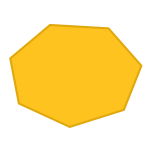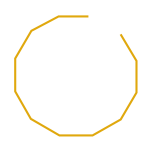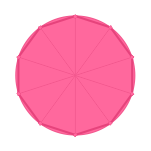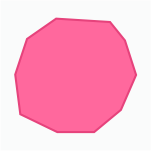# Decagon - Definition with Examples

The Complete K-5 Math Learning Program Built for Your Child

• 40 Million Kids

Loved by kids and parent worldwide

• 50,000 Schools

Trusted by teachers across schools

• Comprehensive Curriculum

Aligned to Common Core

What is Decagon?

A decagon can be defined as a polygon having ten sides, ten interior angles and ten vertices.

Here are some examples of a decagon.Below are non-examples of a decagon.### Types of Decagons:

Decagons can be categorized as regular and irregular.

1. A regular decagon is a decagon with all sides equal in length and all the angles equal in measure. In a regular decagon, the interior angles add up to 1440 degrees, and the exterior angles add up to 360 degrees.

2. An irregular decagon is the type of decagon with unequal sides and angles.

Here is what these decagons look like:REGULAR                   IRREGULAR

 Fun Facts about Decagons:  The word “decagon” dates back to the 16th century, and is derived from the Latin word decagonum, wherein deca means ten and gonum means angles. So, the word decagon means ten angles.

### Decagons in Everyday Life:

Decagons can be commonly seen in various things in the surroundings, such as coasters, coins, umbrellas, drums, watches, cutlery etc.  Also, a decagon is an intriguing form of polygons and is widely used while doing wall art.

Won Numerous Awards & Honors